## CNB13 (PM2) Philosophical Musings – slogans that keep me going

September 19th, 2012

Slogan 1:  EVERYTHING CYCLES

Every (0,1)-pattern, found anywhere in T or C, will repeat, in several (perhaps an infinity of) different directions. And every 0, and every 1, in the pattern, has its repetitions satisfying, or governed by, its own Arithmetic Progression. But this set of APs has one thing in common:  all the APs have the same common difference. There’s an invariance theorem for you!

The music of all these APs, seething and jostling along to eternity, resonating with their joint sounds and common periods of vibration, determines the symphony of the natural numbers. The music of the primes within this cacophony is a main theme, with its very own, basic charms. But, as slogan 2 below says, this theme is controlled, bounded by, and guided by, the patterns of the aforesaid APs.

The single or collective sounds of the primes is certainly not random noise; how can it be, if the humble Arithmetic Progression, with all its magnificent body of chums in every linked pattern, has some say as to when each single prime shall enter the fray, and introduce its own piping, thrumming sound to the orchestration?

Slogan 2:  COPRIMENESS BEGETS PRIMENESS

Every 1 occurring in a (0,1)-pattern signifies a coprimeness between two natural numbers.
Therefore the (0,1)-pattern contains a lot of imformation about the coprimeness, and hence the primeness, of the numbers related to that pattern.

Slogan 3:  POWERS OF PRIMES ARE IMPORTANT

When a prime-sieve (‘p-siv’ for short) is growing in its row of C, it arrives at the leading diagonal (l.d.) at precisely the same moment that a stalactite, its vertical ‘mate’,  is descending (growing) in its vertical column and arrives there too.  At that moment of time a prime cycle-number is born! Let us suppose this happens at the intersection of row Rj and column Cj. So the new prime is p(j) , and the pattern in Rj is 111…1110, its head 0 being on the l.d. This constitutes the fundamental cycle (f.c.) of the cycle-number p(j); the same pattern occurs vertically in Cj, by virtue of neck-tie cycling.

(to be continued)

## CNB12 Formulae for d,e,f,g ; Table of Primorials

September 16th, 2012

In this Blog we shall give formulae for obtaining the frequencies of the 2-vecs d, e, f, and g at the ends of calculations of the cap primorials 22Λ3 , 2Λ3Λ5 , … . We shall index them by k, where p(k) is the kth prime. We also give, first,  a table of primorials for k = 1 to 8, together with values for what we call reduced primorials. We shall use the symbol X(k) (i.e.  CHI-k) for the kth primorial. And X(k, -1), X(k, -2) will denote the first and second reduced primorials respectively, to be explained next.

The first three primorial formulae (on numbers, not cycle-numbers)

The following products are calculated by sequential ordinary multiplication  (i.e. not using  cap products):

Xk    =   pk p(k-1) p(k-2) … p3 p2 p1               ( = pk# )

Xk(-1) =  (pk-1) (p(k-1)­-1) …  (p2-1) (p1-1)    ( = (p­k-1)# )

Xk(-2) =  (pk-2) (p(k-1)­-2) …  (p2-2) (p1-2)    ( = (p­k-2)# )

The first three primorial formulae (on  cycle-numbers)

When computing primorials on cycle-numbers, the same formulae apply, but with cap multiplication. For example,

X =  2’ Λ 3’ Λ 5’  =  30

Table of the Primorials on numbers

 k pk Xk Xk(-1) Xk(-2) 1 2 2 1 1 2 3 6 2 1 3 5 30 8 3 4 7 210 48 15 5 11 2310 480 135 6 13 30030 5760 1485 7 17 510510 92160 22275

Formulae for d, e, f, g

We shall now give formulae for the frequencies of 2-vecs d, e, f, g for two cases: (i) when the cap primorials are calculated from rows of the cycle-number matrix C, and (ii) when the cap primorials are calculated from a matrix called C’ which is derived from C in a manner to be described in a later blog.

Case (i) : calculations from C

d(k) =  X(k-1) – X(k-1 ; -1)  ;

e(k) =  d(k).(p(k) – 1)  ;

f(k)  =  g(k-1)  ;

g(k) =  g(k-1).(p(k) – 1) .      [ Observe that:    g(k)/f(k)  =   e(k)/d(k)  =  (p(k) – 1) . ]

Table of d, e, f, g vakues, for C and k = 1, 2, 3, 4, 5

 k pk – 1 dk ek fk gk 1 1 0 0 1 1 2 2 1 2 1 2 3 4 4 16 2 8 4 6 22 132 8 48 5 10 162 1620 48 480

Case (ii) : calculations from C’

d'(k)  =  2(X(k) – X(k ;  -2))  ;

e'(k)  =   d'(k).(p(k) – 2)  ;

f”(k)  =  2X(k-1 ;  -2) = 2g'(k-1)  ;

g'(k)  =  X(k ;  -2)  =   g'(k-1).(p(k) – 2) .  Observe that:   g'(k)/f'(k)  =  e'(k)/d'(k)  = (1/2). (p(k) – 2) .

Table of d, e, f, g values, for C’ and k = 1  to  5

 k p’k – 1 d’k e’k f’k g’k 1 0 0 0 0 1 2 1 2 1 2 1 3 3 10 15 2 3 4 5 54 135 6 15 5 9 390 1755 30 135

[to be continued, with explanations of C’ ]

## CNB11 Two types of triangle in T (nabla and delta)

September 12th, 2012

Morning (12 Sep. 2012)

This is just a quick Blog. I promise. Early morning thoughts.

I have had you looking for different (0,1)-figures in T already. The following triangle ideas could lead to quite a big study, if anyone would care to attempt it!

We have seen that the neck triangle, of a neck-tie, cycles with the (0,1) pattern of its corresponding cycle-number. What if we studied similar triangles to this one, as they occur all over T? To make it clear what I mean,  first let us define a triangle of type nabla i.e.  one which has ‘tipped-up delta’ shape (here is a delta Δ). I wish I could show you a nabla symbol, but my blog software doesn’t give me one!

The following is a quick general definition of a nabla triangle. Feel free to change it if you find fault, or wish to deal with some other kind of nabla triangle.

Definition 1:  Choose any two points in any  row Rn of T, which have end points labelled 0 or 1.
From those points, and the line segment joining them, form a neck-tie. Call the head of this neck-tie a nabla triangle in T.

Definition 2: A delta triangle Δ in T is a ‘tipped-up’ nabla triangle in T.

Study the two classes of nabla and delta triangles in T. What sort of (0,1)-patterns occur on their boundaries, and in their interiors. Can you set up some kind of algebra for them? Do their patterns and positions tell you anything about the positions of cycle-number f.c.s and prime number patterns? And so on. Endlessly.

What intrigues me, mightily, is that when I make these kinds of study, I know I am doing a mixture of two or three different kinds of mathematics … viz. plane Euclidean geometry in the positive quadrant of a Cartesian frame, things happening with (0,1)-strings (in particular f.c.s of cycle-numbers),  maybe combinatorics, and relationships between the objects concerned (in this case nabla and delta triangles). Every nice pattern, formula or relation which I find is worthy of mathematical joy.  I hope you will find some joy!

It’s all about cycle-numbers. And a slogan COPRIMENESS BEGETS PRIMES!

There! I have kept my promise.  End of Blog.

## CNB10 Multiplication of two cycle-numbers

September 10th, 2012

12 September  2012

A number system would be sadly lacking in interest if you couldn’t ‘do things’ with the  numbers, wouldn’t it? So far I have only shown you how we can look for (0,1)-patterns in triangle T, or in the matrix C. In this Blog I will introduce a way of multiplying two cycle-numbers, say m and n.

This operation has one obvious requirement, that the result shall be a cycle-number (say k) which has its number of (0,1) -symbols equal to mxn. For the new product, we shall write mΛn = k , where Λ (lambda or cap) is the symbol for our new multiplication operation. In the definition of cap, given below, it will be observed that we first work with the fundamental cycles of m and n , to obtain k (the f.c. of k); and then, of course, we cycle it indefinitely to achieve the cycle-number k. Observe also that we shall have occasion to multiply pairs of (0,1)-symbols from within two cycle-numbers, and for this we shall use the same symbol Λ which then follows the multiplication rules:   0Λ0 = 0, 0Λ1 = 0, 1Λ0 = 0, and 1Λ1 = 1. That is a very simple Boolean multiplication table to remember! It follows the same rules as for ordinary multiplication of 0s and 1s.

Definition:  The ‘cap product’ (we sometimes write ‘Boolean Product’, or BP, for this) of two cycle-numbers, is given by the following formula:  (mΛn) = k[n*(m‘)] Λ [m*(n‘)] , where * is the ‘conjoin’ operation for strings. Then cycling of k results in the cycle-number k. The expressions in the square brackets both have length mn, so they can be cap multiplied, taking corresponding elements from the brackets and multiplying them together, using the 0,1 multiplication rules given above (c.f. any element-wise operation on two vectors of equal length).

An example will make the product definition clear.

Let m‘ = (10)’ = 2‘ , and n‘ = (110)’  = 3‘. We shall set  out the cap product operation by writing the first [-] expression from the definition on an upper line, and the second [-] expression directly below it on a lower line. Then we shall compute the element-wise operations on each of the vertical two-vector pairs, thereby obtaining the string which is k

n*2′    =    1 0,1 0,1 0     (3 conjoins of the f.c. of 2 ;  commas  not generally shown)
m*3‘   =    1 1 0, 1 1 0    (2 conjoins of the f.c. of 3  ;  commas  not generally shown)
Λ         =    1 0 0 0 1 0   =  k‘  =  6 .

This looks much like our long multiplication procedure with decimal numbers, doesn’t it? But now everything is much simpler, since we only have to multiply pairs of binary digits, arranged vertically in 2-vecs. Each product yields a result 0 or 1, so there is no ‘carrying’ to be done.

The so-called 2-vecs: In obtaining the final line of this calculation, we applied Λ to six (0,1)– pairs arranged vertically. These pairs are:

1  ,  0  ,  1  ,  0 ,  1  ,  0
1  ,  1  ,  0  ,  1  ,  1  ,  0  .

In order to classify these 2-vecs, we observe that only 4 different such vectors are possible, with the two-element alphabet {0,1}, and we label these by the letters d,e,f,g thus:

Labels:   d = (0,0)’  ;  e = (0,1)’  ;  f = (1,0)’  ;  g = (1,1)’  (here we use the prime symbol to denote vector transposition).

Counting the frequencies of 2-vecs in a product:

Given any pair m, n of cycle-numbers, it is possible to compute the frequencies of the types of 2-vecs in their cap product. (These counts prove to be a very useful tool in a later study of cycle-numbers.)  We shall call these frequencies #d, #e, #f, #g respectively, and give, without proof, the formulae for obtaining them. Thus:  let m0 and m1 be respectively the number of 0s and the number of 1s in m, and similarly let n0 and n1 be respectively the number of 0s and the number of 1s in n‘ : then the required formulae are:
#d = m0xn0 ;  #e = m0xn1 ;  #f = m1xn0  ;  #g = m1xn1 .

N.B. These formulae are attractive and simple, for they follow exactly the pattern of rules given above for the cap product on {0,1}. I gave a general proof  for my cap product of any two (0,1)-strings, in Ref.. It is long and messy!  I am sure there must be a much simpler way of going about it.

To demonstrate, using the example for m = 2 and n = 3 given above, we see that:

for m = 1 0  ,  m0 = 1 and m1 = 1 : whereas for n = 1 1 0 ,  n0 = 1 and n1 = 2 .
Therefore:   #d = m0xn0 = 1 ;  #e = m0xn1 = 2 ;  #f = m1xn0 = 1 ; #g m1xn1 = 2 .

We check this by writing out again the 2-vec table,  and writing the d,e,f,g classifications below each column; then counting the different types. Thus:

1   ,  0  ,   1  ,   0  ,   1   ,   0
1   ,  1  ,   0  ,   1  ,    1   ,   0
g   ,  e  ,   f   ,   e  ,    g   ,    d    …  so there are indeed, one d, two es, one f and two gs.

This Blog contains more than enough material for a blog;  but it is vital stuff for cycle-number theory.

Bye for now.

## CNB9 More about the matrix M(E)

August 28th, 2012

Hi to readers. All zero of them!

Recall from the previous Blog the matrix M(E) of entegers, which I produced from the starting enteger 1v1, using the two operators A(e) and F(e). I said that the Blog diverged from the realm of cycle-numbers, but not very far. Well, this Blog will put that right, and get us back to the cycle-number triangle. In several interesting ways.

Suppose I apply the coprimeness function kappa to each of the elements in M(E). All of the outputs will be 1, since we know that all enteger pairs in our Fibonacci and Arithmetic Progressions are coprime. So the resulting tree of 1s will occur (embed) in matrix C.

Let us take all of these results 1, and find where they occur in the cycle-number triangle κT(E). We shall find that if we place a dot in each of the 1s, and then join up the dots (in well-known play fashion!) we shall get a tree in the domain of T. I will show you the beginnings of this tree in the diagram below.

[to be continued, with the promised diagram]

## CNB7 Enteger Sequences, and a curious AP/FP Pattern Matrix

August 25th, 2012

Good Morning!

This Blog seems to diverge from the topic of  Cycle Numbers. True, but not very far. It will give readers a chance to play mentally with entegers (a simple notion introduced by me in, for example, Schaake, A. G. and Turner, J. C. “The Elements of Enteger Geometry”, Applications of Fibonacci Numbers, Vol. 5, Kluwer A. P., 1993, pp. 569 — 583;  and to puzzle over a Cantorian paradox. I hope you can resolve the paradox for me.

On Enteger Sequences

Given an enteger svt, where s and t are integers. (This should be written as s sub t, but I haven’t learned how to include subscripts using WordPress! An ‘enteger’  just means an ordered pair of integers, arranged on a forward diagonal.)  Addition of two entegers is done as with two vectors. An example enteger is  e = 2v3 (i.e. 2 sub 3) : and an example addition is  2v3 + 3v5 = 5v8 .

We can produce enteger Arithmetic Progressions AP(e), and Fibonacci Progressions FP(e) by use of the following operators:

1. Operator A(svt) ≡ (s+t)v(t) , which produces an enteger AP when applied successively,
2. Operator F(svt) ≡ (t)v(s+t) , which produces an enteger FP when applied successively.

N.B. I think there is a pleasing commutative symmetry in this pair of operators.

An enteger Matrix in E (say M(E))

(Recall that E is the enteger matrix which maps to T , using the kappa function.)

We use the operators A on matrix columns, and B on matrix rows, to produce a doubly-infinite matrix M(E) thus: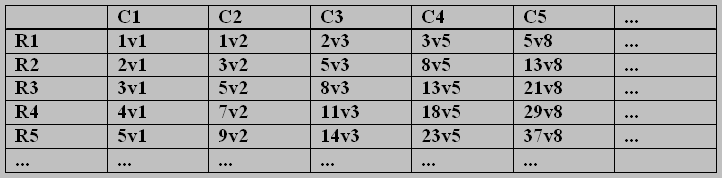The AP/FP Matrix of Entegers (say M(E))

Note that all the column sequences are so-called double-Arithmetic Progressions; whilst all the row aequences are so-called double-Fibonacci Progressions. All these have been obtained by successive operations of A and F respectively, beginning with enteger 1v1.

It is worthy of note that every pair s,t in all cells of M is coprime.  Applying kappa to all pairs changes M into a unit matrix (a U-matrix, with all elements equal to 1).

The next matrix shows the result of removing all the t-values from the entegers, leaving only the integer s-values: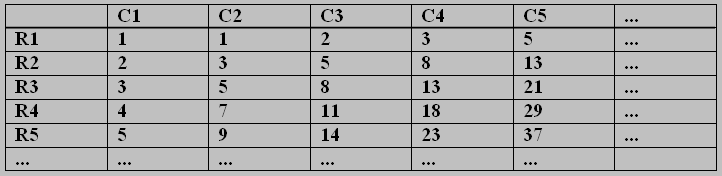The AP/FP Matrix of Integers (say M(I))

The column APs and the row FPs can now be seen clearly as integer sequences, heading off to infinity in the y- and x-directions respectively.

A Curious Paradox on Transfinite Counts

We end this Blog by describing a paradoxical event,  involving counting the number of prime integers in the second matrix. Clearly there are repetitions involved: even in the small table we have shown, there are two 1s, two 2s, three 3s, and four 5s, four 8s etc. (query:  are only Fibonacci numbers repeated?)

It is long known (Dirichlet, 1837) that every A.P.  starting with coprime first and second elements has an inifinity of primes. Whereas it is an open* question whether or not any Fibomacci sequence has an infinity of primes.

*(Note:  Paul Bruckman and I have posted a paper on this very website, claiming to prove that every Fibonacci sequence with coprime starting pair has an infinity of primes; but for over three years the paper has languished in Mathematics Journal Editors’ pending boxes — so far it hasn’t been accepted for publication, although no-one has pointed out any invalid step in the proofs.)

My Paradox, drawn from the matrix, is this:

Suppose I make the hypothesis that ‘no Fibonacci Progression has an infinity of primes’.  Let me call this the Fib. Prog. Primes Conjecture, or FPPC.

Assume that the FPPC is true.   Then:

(i)  Every row in matrix M(I) has a finite number of primes in it;

(ii) Every column in M(I), being an A.P. with coprime starting pair,  has an infinite number of primes (Dirichlet).

Counting the primes from the rows, taken row-by-row, leads to a matching with the list of natural numbers; therefore their total is the first transfinite number  ω,  since the number of rows tends to infinity.

Counting the primes from the columns, each of which posesses an infinity of them, leads to a total of a higher order of infinity (surely so if we consider ordinalities).

This would seem to be an impossibility, since we are counting a multi-set of primes, arranged in a matrix, and counting them  in two different ways.

An alternative viewpoint would be to map all of the primes registered from the Fibonacci rows into the single column C1, an A.P., which contains the countably infinite set of the primes. That leaves all primes in the remaining infinite sequence of columns C2, C3, C4, …, each having an infinity of primes, unmapped by primes from the Fibonacci rows.

I would be grateful if some reader with better knowledge of Cantor’s arithmetic of transfinite numbers would explain to me this seeming paradox.

If, on the other hand, it is not a paradox, then it has demonstrated the falsity of our assumption of the truth of the FPPC. This would mean that at least one Fibonacci sequence has an infinity of primes.  And I believe that from there we can prove that ALL such Fibonacci progressions have an infinity of primes.

End of Blog.

## CNB6 A Night Thought (on serial collapsing)

August 21st, 2012

A  thought for today — I woke up  in the night, and found it on my mind! I haven’t told you yet about some of the details you will need to understand. I’ll very briefly fill you in when I need a particular concept. All very simple.
Serial collapsing (not me):

Every cycle-number is a (0,1) sequence: e.g. 2 = 1010101010 ... I ask what happens to it if you multiply the first element by the second, and write the answer as the start element of a new sequence;  then do the same with the second and third elements; and so on, thereby obtaining a new (0,1)- sequence, because the multiplications  always result in a 0 or a 1.

I have called this product operation a ‘cap product’, or a Boolean Product (BP), and used the symbol Λ, or cap. The product table is: 0Λ0 = 0,  0Λ1 = 0, 1Λ0 = 0. and 1Λ1 = 1. Please learn it for future use in this Blog. It is all over the literature, of course, and I hope I haven’t used a misnomer, or mis-sign. Anyway, it is very useful when studying cycle-numbers, as you will see later. You can get used to handling it now; collapsing 2, thus.

Nearest neighbour collapse (or 1-coll.) of 2 is 1Λ0 = 0 , 0Λ1 = 0, and so on, giving the sequence oooooooo... Not very interesting! Might call it the null sequence; but it is not a cycle-number, is it? Not 0? Remember what 0 is?

Suppose we make the ‘second-nearest-neighbour collapse, or (2-coll.) … which means taking the cap products of each element with the one 2 steps further on in the sequence. That will give us another (0,1)-sequence, which might be a bit more interesting. Thus, collapsing 2 in this way, we get: 2-coll. = 1010101010… .  Surprise? We got back to the original sequence. Then, obviously, if we took this step again, using 3-step collapses on 2, and again using 4-step collapses, we would get nothing new. So the cycle-number 2   ‘has only two serial collapses’. And the last one equals the one you started with!

Next question? How many serial collapses have the cycle-numbers 3, 4, 5, 6?

I’ll leave you to have a go at that one. In particular, look what happens to the series collapses of the prime cycle-numbers. You’ll learn how to use the cap product, which is the major tool in future studies.

## CNB5(PM1) Philosophical Musings (about T, C and the cycle-numbers)

August 17th, 2012

Hi again; second blog of the day!

Just as I was working to finish off CNB4 this morning, I got a grim message, on the screen: my PC couldn’t find the url of my website. Oh dear, thought I!  My son tells me not to worry; and I have already got a grandson-in-law working on the problem. I have faith that they will soon pull it back into the fold.

But that doesn’t stop me from editing a new Blog post,  so I will prepare one giving a few of my thoughts and feelings about the objects which I have called ‘cycle-numbers’. And put down a few comments on why I think they are worth studying.

First let me ask you the old, old, old philosophical question:  “What is Life”.

A good answer occurred to me after I discovered that my website was now only a blank on the screen:      ” A mathematician’s life is one darned problem after another.”

And that is exactly what you will find if you try to prise number secrets out of the (0,1)-patterns you find in T and C: one darned problem after another. And I do hope you are looking, and trying. And that whenever you find one that interests you, that you will try to find relationships with other patterns that you have spotted. Then there are all manner of things that you can try to do; I suppose you might judge that the prize things to obtain is new knowledge about the primes and their distribution amongst the natural numbers. But there is plenty to be said about each of the natural numbers, in general, before trying to discover how the primes arise, and what their roles are in relation to the non-primes (composites). I have indicated a few of such things in earlier blogs; here I just want to say a little about some of the most striking things, which seem to me to lie at the very bottom of the natural number system, from the point of view of my cycle-number representation of it.

(i) It fascinates me that once the alphabet {0,1} is given, and the zero number (0,1)000 … is defined, all that is needed to produce the cycle-number triangle T is to apply the double-cycling method, using the neck-ties, to obtain all the fundamental cycles of the natural numbers in the rows of T.

(ii) I think (poor prejudiced me) that this development of the number system is more basic [just cycling of (0,1)-patterns] than the one obtained from Peano’s axioms. He starts with axiom (1), stating baldly that zero is a number, which contains two undefined terms; then a further four axioms establish an infinite sequence of successors of zero, say {S(0), SS(0), SSS(0), …}, which after lengthy discussion and logical analysis and further definitions, becomes identified with our familiar sequence {1, 2, 3, , …}. And we arrrange the elements of this in a row of gleaming dots in our mind, along our mental number line, which some neurologists now tell us we are born with, to use for comparing group sizes (e.g. groups of lions attacking our tribe!)

(iii) Whereas,  each of my cycle-numbers emerges from its chrysalis in T (or the matrix C), and immediately takes off like a butterfly. fluttering along an infinite line of its own, its (0,1)-pattern growing steadily, in cycles, to infinity. One can imagine it as a complete, though infinite, sequence (I can, anyway!). And I see it also, as carrying a long trail of information for me, about the whole set of natural numbers N. A bit like the early Tiger Moth planes, which flew trailing a long ribbon or banner, advertising some event or other. Not only is the cycle-number in constant motion, pedalling along its own line (i.e. row) in C, but when we look back and forth in its row, we see that it has a past, a present, and a future.

## CNB4 The Cycle-Number Matrix C

August 17th, 2012

Morning!

Now that you are familiar with the cycle-number triangle T, I will show you a matrix which I call C*, which is obtained simply by turning T on its side. You must be familiar with this operation, as it is often done with Pascal’s triangle. It is sometimes (often?) more convenient to study the elements by having them in standard matrix form. To picture C*, you can think of T with a right-angle at its apex, being rotated anti-clockwise through 45 degrees.

Once we have done this, we can imagine our matrix as being an array of elements situated in the Euclidean plane, and refer them to a Cartesian reference frame. This will have Origin at cycle-number zero, and the positive x-axis horizontal as usual through O, whilst the positive y-axis  goes through O but points downwards.  Below are two diagrams, the upper one being T(9), and the lower one being C*. I call C* the augmented C-matrix; it is turned into C by removing  column C0 and row R0. To refer to C‘s elements, move the reference frame so that the origin is in cell (1,1), with no change to the axes except that now x = 1,2,3, … and y = 1,2,3, …

Note that I continue to show the grid lines, but for convenience of reference only. The shown elements (all from {0,1}) are to be thought of as being points in the centres of the grid cells.

Table 1 :  The circle-number triangle T(9)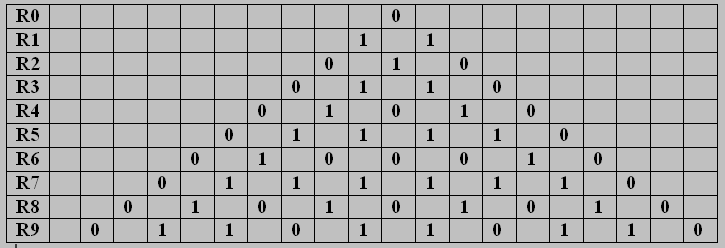You have already done some looking at and studying (0,1)-patterns in triangle T.  So please turn now to the same kinds of study of patterns occurring in matrix C. I will ask a few questions below the table.

Table 2:  The cycle-number matrix C(9, infinity),  seen as a submatrix of C*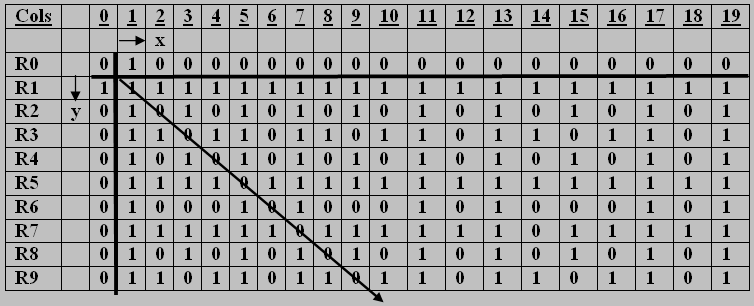Now you can see the start of my cycle-number sequence, with 1 appearing in row R1 , 2 appearing in row R2, and so on. Of course, you can only see bits of them in Table 2, as far as the matrix will show you. By the way, I use a special name for a submatrix of C which starts at the upper left corner of C and has r rows and s columns: I call such submatrices Blocks; so what you can see is Block B(r,s) = B(9, 19). Note the diagonal line, which is the leading diagonal (l.d.) of natrix C.

Now let me ask those promised questions. We can find and study patterns in lines, triangles, squares and so on. As you will learn if you do questions 1 to 5 below.

(q1) What patterns do you find in lines y = 3 , y=6, y=5, y=12, y=13, y=18 ?

(q2) What patterns do you find in lines y=x, y=x+1, y=x+2, y=x+3? How about y=x-1, y=x-2, …?

(q3) Can you find a triangle each of whose sides has pattern 111 ? can you find two such?

(q4) Can you find more than one 111 triangle? Can you find (and prove!)an infinity of them?

(q5) Let us call these equi-side-patterned triangles (espt’s, say). Can you find espt’s with side-patterns 1111, 11111, 111111, … etc. Can you suggest (and prove!) general theorems about this kind of triangle? Let us call them U-figures, since all their elements are units.

(q6) Can you find examples of the following figures in C , and find a few of their properties, in themselves and under cycling for instance.

That will be enough for one Blog! You can think about these questions and figures all of today!

## CNB3 Neck-tie Examples

August 10th, 2012

In CNB2 I showed you the Neck-tie diagram, and described how it is used to construct the rows of the cycle-number triangle T, for rows R1, R2, R3, etc., and asked you to figure-out from my garbled explanation how it is done. To make the matter clear, here are the neck-ties for these first three rows. Mark carefully what they look like and where they come from, and then sketch the next three for yourselves. I use the symbol ni for the ith neck-tie, with i = 1,2,3, …  Of course, we cannot have an n0 neck-tie, because it couldn’t have a neck triangle!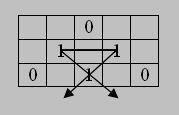Neck-tie n1 for row R1 ; the cycle-number 1 = 111 … You can take this from either the right-leg of the neck-tie, or the left-leg, cycling the 1 downwards.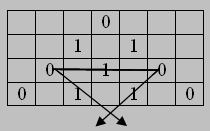Neck-tie n2 for row R2 ; the cycle-number 2 = 101010 … starts from the second element 1 in R2 , then it takes the corner element 0 , making the fundamental cycle 10′ , then it continues cycling down the right-leg towards infinity.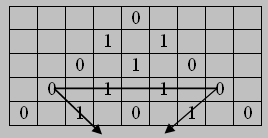Neck-tie n3 for row R3 ; the cycle-number 3 = 110110 … starts from the second element 1 in R3 , then it takes the corner element 0 , making the fundamental cycle 110′ , then it continues cycling down the right-leg towards infinity.

Having made the concept of ‘neck-tie’ clear to you, I hope you will buy a notebook with pages printed with cells in a grid (as shown in my diagrams; usually called a quad book) : and construct a CNT down to, say T(20). You should not show the neck-ties, which were only introduced to define the procedure for row-to-row generation of T, and to help define ‘cycle-number’. Of course, you may omit the grid-lines too. That is easy to do in a print-out, if you have produced your triangle on your PC, perhaps using microsoft WORD (TABLE) or EXCEL.

As you draw your triangle, you will immediately see patterns in the rows and diagonals (and columns) of T.

Two questions, to finish:

Have you tried adding the 1’s in each row? Does the column of sums mean anything to you?

How should we define ‘prime cycle-numbers’?